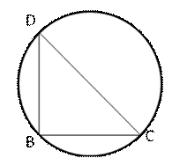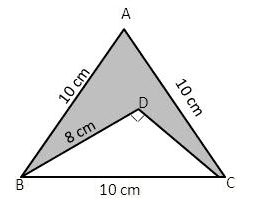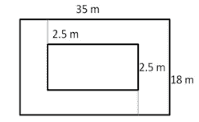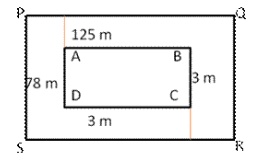RS Aggarwal Class 10 Solutions Chapter 17 Perimeter And Areas Of Plane Figures

RS Aggarwal Class 10 Chapter 17 Perimeter And Areas Of Plane Figures Solutions Free PDF

RS Aggarwal class 10 solutions chapter 17 perimeter and areas of plane figures are provided here. Solve questions from RS Aggarwal to practice the concepts of area and perimeter. In the previous classes, students were taught the basic concepts of area and plane figures. In class 10 students will learn how to find the area of the combination of plane figures.

Q.1: Find the area of the triangle whose base measures 24 cm and the corresponding height measures 14.5 cm.

Sol:

Area of given triangle = $\frac{1}{2}\times Base\times Height$ = $(\frac{1}{2}\times 24\times 14.5)cm^{2}=174cm^{2}$

Q.2: Find the area of the triangle whose sides are 42 cm, 34 cm and 20 cm. Also, find the height corresponding to the longest side.

Sol: Let a = 42cm, b = 34cm and c = 20cm

Then, s = $\frac{1}{2}(42+34+20)cm$ = 48cm

(s – a) = 6cm, (s – b) = 14cm and (s – c) = 28cm

(i) Area of triangle = $\sqrt{s(s-a)(s-b)(s-c)}$ = $\sqrt{48\times 6\times 14\times 28}\;cm^{2}=336\;cm^{2}$

(ii) Let base = 42 cm and corresponding Height = h cm

Then area of triangle = $(\frac{1}{2}\times 42\times h)cm^{2}=(21h)cm^{2}$

21h = 336 i.e. h = $\frac{336}{21}=16cm$

Hence, the height corresponding to the longest side = 16cm

Q.3: Find the area of the triangle whose sides are 18 cm, 24 cm and 30 cm. Also, find the height of the corresponding to the smallest side.

Sol: Let a = 18cm, b = 24cm, c = 30cm

Then, 2s = (18 + 24 + 30) cm = 72 cm

s = 36cm

(s – a) = 18cm, (s – b) = 12cm and (s – c) = 6cm

(i) Area of triangle = $\sqrt{s(s-a)(s-b)(s-c)}$ = $\sqrt{36\times 18\times 12\times 6}\;cm^{2}$ = $216\;cm^{2}$

(ii) Let base = 18 cm and altitude = x cm

Then, area of triangle = $(\frac{1}{2}\times 18\times x)=9x\;cm^{2}$

9 x = 216 x = $\frac{216}{9}$ = 24

Hence, altitude corresponding to the smallest side = 24 cm

Q.5: The sides of the triangle are in the ratio 5 : 12 : 13 , and it perimeter is 150 m. Find the area of the triangle.

Sol: On dividing 150m in the ratio 5 : 12 : 13, we get

Length of one side = $(150\times \frac{5}{30})m=25m$

Length of the second side = $(150\times \frac{12}{30})m=60m$

Length of third side = $(150\times \frac{13}{30})m=65m$

Let a = 25m, b = 60m, c = 65m

Then, s = $\frac{1}{2}(25+60+65)m=75m$

(s – a) = 50cm, (s – b) = 15cm, and (s – c) = 10cm

Area of the triangle = $\sqrt{s(s-a)(s-b)(s-c)}$ = $\sqrt{75\times 50\times 15\times 10}\;m^{2}$ = $750\;m^{2}$

Hence, area of the triangle = $750\;m^{2}$

Q.6: The perimeter of the triangular field is 540m, and its sides are in the ratio 25 : 17 : 12. Find the area of the field. Also find the cost of plugging the field at Rs. 40 per 100 $m^{2}$.

Sol: On dividing 540m in ratio 25 : 17 : 12, we get

Length of one side = $(540\times \frac{25}{54})m=250m$

Length of second side = $(540\times \frac{17}{54})m=170m$

Length of third side = $(540\times \frac{12}{54})m=120m$

Let a = 250m, b = 170m and c = 120m

Then, s = $\frac{1}{2}(250+170+120)m=270m$

Then, (s – a) = 29m, (s – b) = 100m, and (s – c) = 150m

Area of the triangle = $\sqrt{s(s-a)(s-b)(s-c)}$

= $\sqrt{270\times 29\times 100\times 150}\;m^{2}$ = $9000\;m^{2}$

The cost of plugging 100 area is = Rs. 18.80

The cost of plugging 1 is Rs. $\frac{18.80}{100}$

The cost of plugging 9000 area = Rs. $(\frac{18.80}{100}\times 9000)$ = Rs. 1692

Hence, area of the triangle = $60cm^{2}$

Q.7: The difference between the sides at right angles in right angled triangle is 7 cm. The area of the triangle is 60 $cm^{2}$.Find its perimeter.

Sol: Let the sides containing the right angle be x cm and (x – 7) cm

Then, its area = [1/2 * x * (x-7)] $cm^{2}$

But, area = 60 $cm^{2}$

Therefore, 1/2 * (x-7) = 60

$x^{2}-7x-120=0$

i.e $x^{2}-15x+8x-120=0$

x(x – 15) + 8(x – 15) = 0

(x – 15)(x + 8) = 0

x = 15[Neglecting x = -8]

One side = 15cm and other = (15 * 7) cm = 8 cm

Hypotenuse = $\sqrt{(15)^{2}+(8)^{2}}cm$ = $\sqrt{225+64}cm$

$\sqrt{289}cm$ = 17 cm

Perimeter of triangle (15 + 8 + 17) cm = 40 cm

Q.8: The length of the two sides of a right triangle containing the right angle differ by 2 cm. If the area of the triangle is 24 $cm^{2}$ , find the perimeter of the triangle.

Sol: let the sides containing the right angle be x and (x – 2) cm

Then, its area = (1/2 * x * (x – 2)) $cm^{2}$

But area = 24$cm^{2}$

Therefore, 1/2 * (x – 2) = 24

$x^{2}-2x-48=0$

i.e. $x^{2}-8x+6x-48=0$

x(x – 8) + 6(x – 8) =0

(x – 8)(x + 6) = 0

x = 8 [Neglecting x = -6]

One side = 8cm, and other (8 * 2) cm = 6cm

Hypotenuse = $\sqrt{(8)^{2}+(6)^{2}}cm$ = $\sqrt{64+36}cm$ = $\sqrt{100}cm$

Therefore, perimeter of the triangle = 8 + 6 +10 = 24 cm

Q.9: Each side of the equilateral triangle is 10cm. Find (i) the area of the triangle and (ii) the height of the triangle.

Sol: Here a = 10 cm

Area of the triangle = $(\frac{\sqrt{3}}{4}\times a^{2})$ SQ.. unit

= $(\frac{\sqrt{3}}{4}\times 10\times 10)=43.3cm^{2}$

(ii) Height of the triangle = $(\frac{\sqrt{3}}{4}\times a)$ SQ.. units

= $(\frac{\sqrt{3}}{4}\times 10)=4.3cm$

Hence, area = 43.3cm2 and height = 4.3 cm

Q.10: The height of an equilateral triangle is 6 cm. Find its area. Take $\sqrt{3} = 1.73$.

Sol: Let the sides of the equilateral triangle be x cm.

As, the area of an equilateral triangle = $\frac{\sqrt{3}}{4} \; (side)^{2} = \frac{x^{2}\sqrt{3}}{4}$

Also, the area of the triangle = $\frac{1}{2}\times base\times height = \frac{1}{2}\times x\times 6 = 3x$

$\frac{x^{2}\sqrt{3}}{4}= 3x$

$\frac{x \sqrt{3}}{4}= 3$

$x= \frac{12}{\sqrt{3}}$

$x= \frac{12}{\sqrt{3}}\times \frac{\sqrt{3}}{\sqrt{3}}$

$x= \frac{12 \sqrt{3}}{3} = 4\sqrt{3}$ cm

Now, area of the equilateral triangle = 3x

= $x= 3 \times 4\sqrt{3}$ cm

$= 12\sqrt{3}$ cm

$= 12 \times 1.73$ = $20.76 cm^{2}$

Q.11: If the area of an equilateral triangle is $36\sqrt{3}$ , find its height.

Sol: Let each side of the equilateral triangle be a cm

Then, its area = $(\frac{\sqrt{3}}{4}\times a^{2})$ cm

Therefore, $(\frac{\sqrt{3}}{4}\times a^{2})$ = $36\sqrt{3}\Rightarrow a^{2}=(\frac{36\sqrt{3}\times 4}{\sqrt{3}})=144$

a = 12 cm

Perimeter of equilateral triangle = 3a = (3 * 12) cm = 36 cm.

Q.12: If the area of an equilateral triangle is $81\sqrt{3}$, find its height.

Sol: Let each side of the equilateral triangle be a cm

Area of equilateral triangle = $(\frac{\sqrt{3}}{4}\times a^{2})$ cm

$(\frac{\sqrt{3}}{4}\times a^{2})$ = $81\sqrt{3}\Rightarrow a^{2}(\frac{81\sqrt{3}\times 4}{\sqrt{3}})=324$

a = $\sqrt{324}$ = 18cm

Height of equilateral triangle = $\frac{\sqrt{3}}{2}a=(\frac{\sqrt{3}}{2}\times 18)cm=9\sqrt{3}cm$

Q.13: The base of a right angled triangle measures 48 cm and its hypotenuse measures 50 cm. find the area of the triangle.

Sol: Base of right angled triangle = 48 cm

Height of the right angled triangle = $\sqrt{(hypotenuse)^{2}-(base)^{2}}$

Height = $\sqrt{(50)^{2}-(48)^{2}}cm$ = $\sqrt{2500-2304}cm$ = $\sqrt{196}cm$ = 14cm

Area of triangle = $(\frac{1}{2}\times 48\times 14)cm^{2}$ = $336\;cm^{2}$

Q.14: The hypotenuse of a right-angled triangle is 65 cm and its base is 60cm. Find the length of perpendicular and the area of the tringle.

Sol:

Hypotenuse = 65 cm

Base = 60 cm

In a right-angled triangle,

$(Hypotenuse)^{2} = (Base)^{2} + (Perpendicular)^{2}$

$(65)^{2} = (60)^{2} + (Perpendicular)^{2}$

$(Perpendicular)^{2} = (65)^{2} – (60)^{2}$

$(Perpendicular)^{2} = (65 – 60 )(65 + 60)$

$(Perpendicular)^{2} = 5 \times 125$

$(Perpendicular)^{2} = 625$

$(Perpendicular) = 25$

Area of triangle = $\frac{1}{2} \times base \times perpendicular$

$\frac{1}{2} \times 60 \times 25$

$= 750 cm^{2}$

Q.15: Find the area of a right-angled triangle, the radius of whose circumcircle measures 8cm and the altitude drawn to the hypotenuse measures 6cm.

Sol: The circumference of a right-angled triangle is the midpoint of the hypotenuseHypotenuse = 2 * (radius of circumcircle) = (2 * 8) cm = 16 cm

Base = 16 cm, height = 6 cm

Area of right angled triangle = (1/2 * base * height) = (1/2 * 16 * 6) cm = $48\;cm^{2}$

Q.16: Find the length of the hypotenuse of an isosceles right angled triangle whose area is $200\;cm^{2}$ . Also find its perimeter.

Sol: Let each eQ.ual side be a cm in length.

Then,

1/2 * a * a =200 → a = 20 cm

Hypotenuse (h) = $\sqrt{a^{2}+a^{2}}\;cm$

= $a\sqrt{2}cm=20\sqrt{2}cm$ = (20 * 1.414) cm = 28.28 cm

Therefore, Perimeter of the triangle = (2a = h) cm = (2 * 20 + 28.28) cm =68.28 cm

Hence, hypotenuse =28.28 cm and perimeter = 68.28 cm

Q.17: The base of an isosceles triangle measures 80 cm and its area is $360\;cm^{2}$.Find the perimeter of the triangle.

Sol: Let each eQ.ual side be ‘a’ cm and base = 80 cm

Area = $\frac{1}{4}b\times \sqrt{4a^{2}-b^{2}}$ square units

=$\frac{1}{4}\times 80\times \sqrt{4a^{2}-6400}\;cm^{2}$ = $20\times \sqrt{4a^{2}-6400}\;cm^{2}$

But area = $360\;cm^{2}$

Therefore, $20\sqrt{4a^{2}-6400}=360$

$20\times 2\sqrt{a^{2}-1600}=360$

$\sqrt{a^{2}-1600}=9$

$a^{2}-1600=81$

$a^{2}=1681$

Therefore, a = 41 cm

Perimeter of triangle = (2a + b) cm

= (2 * 41 + 80) cm = (82 + 80) cm = 162 cm

Hence, perimeter of the triangle = 162 cm

Q.18: Each of the eQ.ual sides isosceles triangle measures 2 cm more than its height, and the base of the triangle measures 12 cm. Find the area of the triangle?

Sol: Let the height be h cm, then a = (h + 2) cm and b = 12 cm

1/2 *12*h = 1/4 * 12 * $\sqrt{4(h+2)^{2}-144}$

6h = $6\sqrt{(h+2)^{2}-36}$

h = $\sqrt{(h+2)^{2}-36}$

On squaring both sides:

$h^{2}=(h+2)^{2}-36$

$h^{2}=h^{2}+4+4h-36$

-4h = -32 i.e. h = 8cm

Therefore, a = h+2 = (8+2)cm = 10 cm

Area of isosceles triangle = $\frac{1}{4}b\times \sqrt{4a^{2}-b^{2}}$

$\frac{1}{4}\times 12\times \sqrt{4\times (10)^{2}-(12)^{2}}$

= $3\sqrt{400-144}=3\times \sqrt{256}$ = 3 * 16 = 48 $cm^{2}$

Hence, area of the triangle = 48 $cm^{2}$

Q.19: Find the area and perimeter of an isosceles right triangle, each of whose eQ.ual sides measures 10cm.

Sol: Let $\bigtriangleup ABC$ is a isosceles triangle. Let AC, BC be the equal sides

Then AC = BC = 10cm. Let AB be the base of $\bigtriangleup ABC$ right angle at C.

AB = $\sqrt{AC^{2}+BC^{2}}=\sqrt{(10)^{2}+(10)^{2}}\;cm^{2}$

= $\sqrt{20}cm=10\sqrt{2}cm$

Perimeter = (2a + b) square units

= (2*10 + $10\sqrt{2}$)cm = (20 +10 *1.414)cm = (20 + 14.14) cm = 34.14 cm

Area of isosceles triangle ABC

= 1/2 * 10 *10 $cm^{2}$ = 50 $cm^{2}$

Hence, the area = 50 $cm^{2}$ and perimeter = 34.14 cm

Q.20: In the given figure, $\bigtriangleup ABC$ is an equilateral triangle the length of whose side is eQ.ual to 10 cm, and $\bigtriangleup DBC$ is the right angled triangle at D and BD = 8cm. Find the area of the shaded region.Sol: Area of shaded region = Area of $\bigtriangleup ABC$ – Area of $\bigtriangleup DBC$

First we find area of $\bigtriangleup ABC$

Therefore, Area = $\frac{\sqrt{3}}{4}a^{2}=(\frac{\sqrt{3}}{4}\times 10\times 10)cm^{2}$ = 43.30 $cm^{2}$

Second we find area of $\bigtriangleup DBC$ which is right angled

Therefore area of triangle DBC = 1/2 * Base * Height

Height = $\sqrt{BC^{2}-DB^{2}}=\sqrt{10^{2}-8^{2}}$ = $\sqrt{100-64}=\sqrt{36}=6\;cm$

Therefore, Area = 1/2  *DB *DC = (1/2  * 8 * 6)  $cm^{2}$ = 24 $cm^{2}$

Area of shaded region = Area of $\bigtriangleup ABC$ – Area of $\bigtriangleup DBC$ = (43.30 – 24) = 19.30

Area of shaded region = 19.3

Exercise – 17B

Q.1: The perimeter of a rectangular plot of land is 80 m and its breadth is 16 m. find the length and area of the plot.

Sol: Let the length of plot be x meters Its perimeter = 2 [length + breadth]

= 2(x + 16) = (2x +32) meters

Therefore, (2x + 32) = 75 i.e. 2x = 75 – 32

2x = 43 i.e. x = 43/2 = 21.5

Length of the rectangle is 21.5 meter

Area of the rectangular plot = length * breadth = (16 * 21.5) $m^{2}$

= 344 $m^{2}$

The length = 21.5 m and the area = 344 $m^{2}$

Q.2: The length of a rectangular park is twice its breadth and its perimeter is 840. Find the area of the park.

Sol: Let the breadth of a rectangular park be x meters

Then, its length = 2x meter

Therefore, perimeter = 2(length + breadth) = 2(2x + x) = 6x meters

Therefore, 6x = 840m [1km = 1000m]

i.e x = 140m

Then, breadth = 140m and length = 280m

Area of rectangular park = (length * breadth) = (140 * 280) $m^{2}$

= 39200 $m^{2}$

Hence, area of the park = 39200 $m^{2}$

Q.3: One side of a rectangle is 12cm long and its diagonal measures 37cm. Find the other side and the area of the rectangle.

Sol: Let ABCD be the rectangle in which in which AB =12cm and AC = 37cmBy Pythagoras theorem, we have BC = $\sqrt{AC^{2}-AB^{2}}$ units

= $\sqrt{(37)^{2}-(12)^{2}}\;cm^{2}$ = $\sqrt{(37+12)(37-12)}\;cm$ = $\sqrt{49\times 25}\;cm$ = $\sqrt{1225}\;cm$ = 35 cm

Thus, length = 35 cm and breadth = 12cm

Area of rectangle = (12 * 35) $cm^{2}$ = 420 $cm^{2}$

Q.4: The area of a rectangular plot is 462 $m^{2}$ and its length is 28 m. Find its perimeter.

Sol: Let the breadth of the plot be x meter

Area = Length * Breadth = (28 * x) meter

= 28x $m^{2}$

Therefore, 28x = 462 i.e. x = 462/28 = 16.5 m

Breadth of plot is = 16.5 m

Perimeter of the plot is 2(length + breadth) = 2(28 + 16.5) m = 2 (44.5) m = 89 m

Q.5: A lawn is in the form of a rectangle whose sides are in the ratio 5 : 3. The area of the lawn is 3375 $m^{2}$ . Find the cost of fencing the lawn at Rs. 65 per meter.

Sol: Let the length of lawn be 5x m and breadth of the lawn be 3x m

Area of rectangular lawn = (5x * 3x) $m^{2}$ =

($15x^{2}$ ) $m^{2}$

Area of lawn = 3375 $m^{2}$

$15x^{2}=3375\Rightarrow x^{2}=\frac{3375}{15}=225$

$x=\sqrt{225}$ =15 m

Length = 5 * 15 = 75

Breadth = (3 *15)m = 45 m

Perimeter of lawn = 2(length + breadth) = 2(75 +45)m =240 m

Cost of fencing the lawn per meter = Rs. 8.50 per meter

Cost of fencing the lawn = Rs. 8.50 * 240 = Rs. 2040

Q.6: A room is 16m long and 13.5m broad. Find the cost of covering its floor with 75m wide carpet at Rs. 60 per meter.

Sol: Length of the floor = 16 m

Breadth of the floor = 13.5 m

Area of floor = (16 * 13.5) $m^{2}$

Length of the carpet = $\frac{Area\;of\;floor}{width\;of the\;carpet}$ =$\frac{16\times 13.5}{0.75}m=288m$

Cost of carpet = Rs. 15 per meter

Cost of 288 meters of carpet = Rs. (15 * 288) = Rs. 4320

Q.7: The floor of a rectangular hall is 24 m long 18m wide. How many carpets, each of the 2.5m and breadth 80cm, will be required to cover the floor of the hall?

Sol: Area of floor = length * breadth = (24 * 18) $m^{2}$

Area of carpet = Length * breadth = (2.5 * 0.8) $m^{2}$

Number of carpets = $\frac{Area\;of\;floor}{Area\;of\;carpet}=\frac{(24\times 18)m^{2}}{(2.5\times 0.8)m^{2}}$ = 216

Hence the number of carpet pieces required = 216

Q.8: A 36m long, 15m broad verandah is to be paved with stones, each measuring 6dm by 5dm. How many stones will be required?

Sol: Area of verandah = (36 * 15) $m^{2}$ = 540 $m^{2}$

Area of stone = (0.6 * 0.5) $m^{2}$ [10dm = 1m]

Number of atones required = $\frac{Area\;of\;verandah}{Area\;of\;stone}=\frac{540}{0.3}=1800$

Hence, 1800 stones are required to pave the verandah.

Q.9: The area of a rectangle is 192$cm^{2}$ and its perimeter is 56cm. Find the dimension of the rectangle.

Sol: Perimeter of rectangle = 2(l + b)

2(l + b) = 56 i.e. l + b = 28cm

b = (28 – l) cm

Area of rectangle = 192 $cm^{2}$

l * (28 – l) = 192

28l – $l^{2}$ = 192

$l^{2}-28l+192=0$

$l^{2}-16l-12l+192=0$

l(l – 16) – 12(l – 16) = 0

(l – 16)(l – 12) = 0

l = 16 cm or l = 12

Therefore, length = 16cm and breadth = 12cm

Q.10: A rectangular park 35m long and 18m wide is to be covered with grass, leaving 2.5m uncovered all around it. Find the area to be laid with grass.

Sol: Length of the park = 35 m

The breadth of the park = 18 mArea of the park = (35 * 18) $m^{2}$ = 630 $m^{2}$

Length of the park with grass = (35 – 5) = 30 m

Breadth of the park with grass = (18 – 5) m = 13 m

Area of park with grass = (30 * 13) $m^{2}$ =390 $m^{2}$

Area of path without grass = area of the whole park – area of park with grass = 630 – 390 = 240 $m^{2}$

Hence, area of the park to be laid with grass = 240 $m^{2}$

Q.11: A rectangular plot measures 125m by 78 m. It has a gravel path 3m wide all around on the outside. Find the area of the area of the path and the cost of gravelling it at Rs. 75 per $m^{2}$.

Sol: Length of the plot = 125 m

The breadth of the plot = 78 mArea of plot ABCD = (125 * 78) $m^{2}$ = 9750 $m^{2}$

Length of the plot including the path = (125 + 3 + 3) m = 131 m

Breadth of the plot including the path = (78 + 3 + 3) m = 84 m

Area of plot PQRS including the path = (131 * 84) $m^{2}$ = 11004 $m^{2}$

Area of path = Area of plot PQRS – Area of plot ABCD

= (11004 – 9750) $m^{2}$ = 1254 $m^{2}$

Cost of gravelling = Rs. 75 per $m^{2}$

Cost of gravelling the whole path = Rs. (1254 * 75) = Rs. 94050

Hence, cost of gravelling the path = Rs. 94050

Access CBSE Sample Paper for class 10 Maths here.

Access NCERT Book for class 10 Maths here.

Practise This Question

Above picture is a bi-focal lens, used to correct a presbyopic person, what is region 1 and 2?